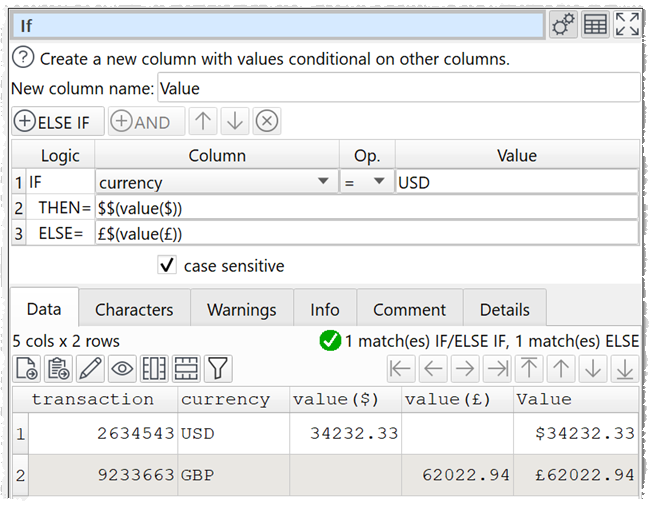﻿ Reference > Column variables

# Column variables

Transforms such as If, Filter, Insert, Replace and Substitute allow you to use the values of columns on the same row using column variables. Column values can be referenced either:

By column header name, e.g. \$(item cost) for the 'item cost' column; or

By column index, e.g. \$(1) for the first column.

Notes:

The column name is case sensitive. E.g. column 'A' is referenced by variable \$(A) and column 'a' is referenced by variable \$(a).

Whitespace at the start or end of the column name is ignored. E.g. column ' A ' is referenced by variable \$(A).

Whitespace within the column variable is important. E.g. column 'AB' is not referenced by variables \$(A B), \$ (AB) \$( AB) or \$(AB ).

If multiple columns have the same name, the first column from the left with that name will be used.

Reference by name takes priority over reference by index. For example, if there is a column named "1" then \$(1) will refer to that rather than the first column.

Column variables are replaced as text. For example if column 'A' has value '2' and column 'B' has value '3', then \$(A)*\$(B) evaluates as '2*3' (not '6').

Column names can contain \$, ( and ) characters. For example: